Chestnuts

Neil has 5-times chestnuts less than Adrian. Together they have 894 chestnuts.

How many chestnuts has Neil and Adrian?

Result

Neil:  149

Solution:Leave us a comment of example and its solution (i.e. if it is still somewhat unclear...):Be the first to comment!To solve this verbal math problem are needed these knowledge from mathematics:

Do you have a linear equation or system of equations and looking for its solution? Or do you have quadratic equation?

Next similar examples:

1. Isosceles trapezoidPerimeter of the isosceles trapezoid is 48 cm. One side is two times greater than the second side. Determine the dimensions of the trapezoid.
2. Number unknownAdela thought the two-digit number, she added it to its ten times and got 407. What number does she think?
3. Ball gameRichard, Denis and Denise together scored 932 goals. Denis scored 4 goals over Denise but Denis scored 24 goals less than Richard. Determine the number of goals for each player.
4. BenchesThe park has 64 benches. Occupied are by 18 more than empty. How many benches are occupied and empty ?
5. Book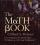John read the book so that it read 30 pages per day. Maxim read 34 pages a day and read it two days earlier than John. How many pages has the book?
6. ProductResult of the product of the numbers 1, 2, 3, 1, 2, 0 is:
7. Expression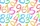If A=2 B=3 evaluate expression A(B+A) and multiply it by A
8. Unknown number xyzFind the number that its triple is 24. Solve by equation.
9. If-then equationIf 5x - 17 = -x + 7, then x =
10. Hotel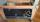The rooms in the mountain hotel are double and triple. Double rooms are 25 and triple are 17 more. How many rooms are there in this hotel?
11. What isWhat is the value of the smaller of a pair of numbers for which their sum is 78 and their division quotients are 0.3?
12. Computer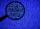A line of print on a computer contains 64 characters (letters, spacers or other chars). Find how many characters there are in 7 lines.
13. Decimal expansion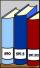Calculate: 2 . 1 + 0 . 10 + 7 . 10000 + 4 . 1000 + 6 . 100 + 0 . 100000 =
14. I thinkI think a number. When I multiply it by five, and after that I subtract 477, I get the same number as if I multiplied it twice. What number do I think?
15. Football match 4In a football match with the Italy lost 3 goals with Germans. Totally fell 5 goals in the match. Determine the number of goals of Italy and Germany.
16. Icecreams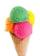Karthik bought 30 icecreams for his birthday .2/5 of them are chocolate icecreams. Find the number of chocolate icecreams he bought?
17. Eq1Solve equation: 4(a-3)=3(2a-5)